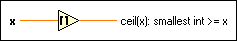# Round Toward +Infinity Function

## LabVIEW 2018 Help

Edition Date: March 2018
Part Number: 371361R-01
View Product InfoLabVIEW 2016 HelpLabVIEW 2017 HelpLabVIEW 2018 HelpLabVIEW 2019 HelpLabVIEW 2020 Help

Owning Palette: Numeric Functions

Requires: Base Development System

Rounds the input to the next highest integer.

For example, if the input is 3.1, the result is 4. If the input is –3.1, the result is –3. The connector pane displays the default data types for this polymorphic function.

Examplex can be a scalar number, array or cluster of numbers, array of clusters of numbers, and so on.ceil(x): smallest int >= x is the resulting highest integer, closest to x. If the input is a time stamp value, the function rounds to the next second. When x is of the form x = a + bi, that is, when x is complex, the function returns a complex number defined by the next highest integers to the real and imaginary parts of x. The following equation defines ceil(x): smallest int >= x: ceil(x) = ceil(a) + i ceil(b)

## Example

Refer to the Numeric Functions VI in the labview\examples\Numerics directory for an example of using the Round Toward +Infinity function.